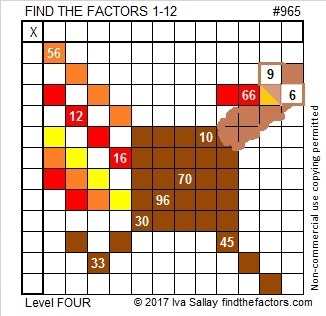# 965 Run, Turkey, Run!

Happy Thanksgiving, everyone!

I didn’t mean to make any Thanksgiving puzzles but after I created the puzzles this week, I could see some Thanksgiving-related pictures in the designs I had already made. This one is my favorite.

Run, Turkey, Run! For millions of turkeys today, it’s already too late.Print the puzzles or type the solution in this excel file: 12 factors 959-967

Here is a little about the number 965:

965 is the sum of two squares two different ways:
26² + 17² = 965
31² + 2² = 965

So it is also the hypotenuse of FOUR Pythagorean triples, two of them primitives:
124-957-965, calculated from 2(31)(2), 31² – 2², 31² + 2²
387-884-965, calculated from 26² – 17², 2(26)(17), 26² + 17²
475-840-965 which is 5 times (95-168-193)
579-772-965 which is (3-4-5) times 193

• 965 is a composite number.
• Prime factorization: 965 = 5 × 193
• The exponents in the prime factorization are 1 and 1. Adding one to each and multiplying we get (1 + 1)(1 + 1) = 2 × 2 = 4. Therefore 965 has exactly 4 factors.
• Factors of 965: 1, 5, 193, 965
• Factor pairs: 965 = 1 × 965 or 5 × 193
• 965 has no square factors that allow its square root to be simplified. √965 ≈ 31.064449